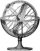SPIP

# Article validated

The article « {where} (https://www.spip.net/en_article6734.html) » was
validated by George.

## {where}

Tuesday 23 August 2022 , by jack

In some cases, SPIP’s specific criteria do not allow a complex request to
be described. Loops can use the `{where}` criterion to directly specify the
WHERE of the SQL query.

The where criterion expects a single argument, which is the conditional
part of a (MY)SQL query. SPIP uses this value by combining it with the
other conditional elements from the other criteria in the loop.

**Examples **

The where criterion allows ORs to be performed:

``````<BOUCLE_or

(ARTICLES)

{statut=redac}

{where id_rubrique=10 OR id_rubrique=20}

>
``````

It allows you to call MYSQL functions:

``````<BOUCLE_or

(ARTICLES)

{where YEAR(date_creation) > 1970}

>
``````

It allows you to make subqueries (here in the plug-in `info_site`) :
`{where id_organisation IN (SELECT id_objet FROM spip_projets_liens WHERE objet='organisation' AND id_projet=#ID_PROJET)}`

The where criterion makes it possible to use a condition where the 2
terms of the test are fields of the table: we want to find the articles
with the same section id and sector id

``````<BOUCLE_where

(ARTICLES)

{where id_rubrique = id_secteur}

>
``````

Note that it would have been possible to do it without where in all
these cases:
with `{id_rubrique IN #LIST{10,20}}`
with `{date_creation>1970-01-01}`
with a joint in the SPIP loop
with a wrapping loop

Preparing the condition
When there is a comma in the where expression, often because of a MYSQL
function call, include the condition in a `#VAL` tag.
Example :
`{where #VAL{"DATE_ADD(date_debut,INTERVAL 2 WEEK)<date_fin"}}`

When the conditions are even more complex, it is necessary to prepare the
query in a `#SET` :

``````#SET

{where, here complex calculation}

<BOUCLE_root_articles

(ARTICLES)

{...}

{where

#GET

{where}

}

>
``````

Conditional where criterion

The criterion also allows comparison with the value of `where` passed into
the environment, if it exists: `{where?}`

— Sent by SPIP (https://www.spip.net/)